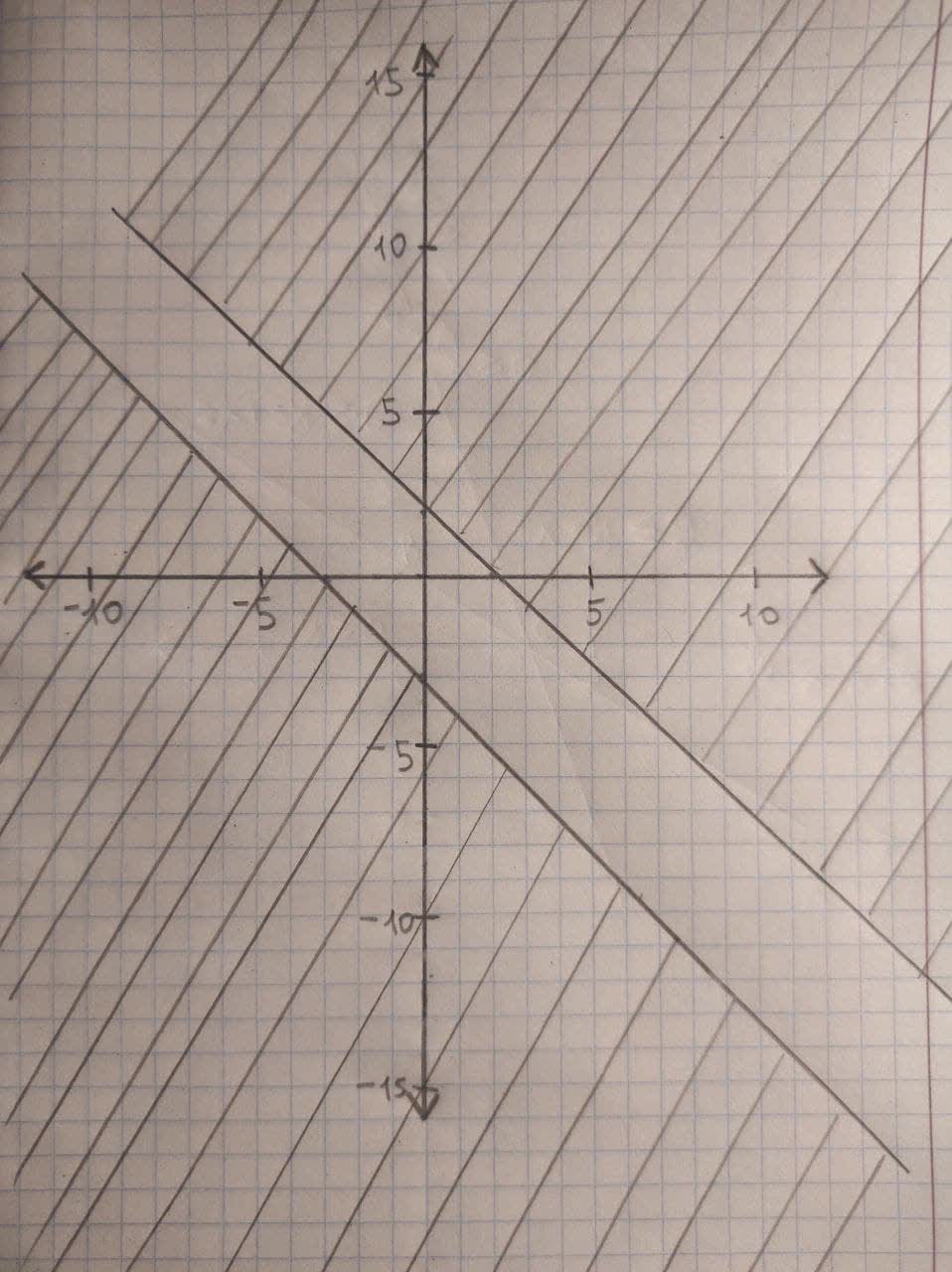#### Didn’t find what you are looking for?

Question# Graph the solution set of the system of inequalities:x+y>2x+y<-3

Inequalities systems and graphs
ANSWEREDGraph the solution set of the system of inequalities:
$$x+y>2$$
$$x+y<-3$$2021-01-14

Graph of the solution of the inequality $$x+y>2$$ is the region above the line $$x+y=2$$. The points on the line x+y=2 are not solutions of the inequality as it is a strict inequality.
So we draw a dotted line for the line $$x+y=2.$$
Similarly, the graph of the solution of the inequality $$x+y<−3$$ is the region below the line $$x+y=−3$$. The points on the line $$x+y=−3$$ are not solutions of the inequality as it is a strict inequality.
So we draw a dotted line for the line $$x+y=−3$$.